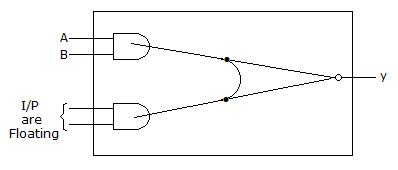# Electronics and Communication Engineering - Digital Electronics

21.

In 8085 microprocessor, if instruction RST is written in a program then the program will jump to location

 A. 0020 H B. 0024 H C. 0028 H D. 002 CH

Explanation:

No answer description available for this question. Let us discuss.

22.

figure given below shows the internal schematic of a TTL AND-OR Invert (AOI) gate, For the input shown in the given figure, the output Y isA. 0 B. 1 C. AB D. AB

Explanation:

No answer description available for this question. Let us discuss.

23.

In 8085 microprocessor, the RST 5.5 interrupt service routine start from location

 A. 0020 H B. 0024 H C. 0028 H D. 002 CH

Explanation:

No answer description available for this question. Let us discuss.

24.

Which memory requires periodic recharging?

 A. All ROMS B. All RAMS C. Static RAM D. Dynamic RAM

Explanation:

No answer description available for this question. Let us discuss.

25.

The hexadecimal number given below represent decimal numbers which are multiples of DC, C8, BE, 46, IE

 A. 2 B. 6 C. 7 D. 10